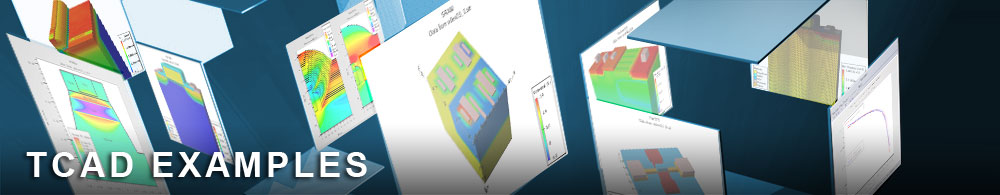Noise from a MOSFET

## noiseex03.in : Noise from a MOSFET

Requires: S-Pisces/Noise
Minimum Versions: Atlas 5.28.1.R

This example investigates the noise generated by a two-port device.

A silicon MOSFET is modeled and a DC-IV sweep is performed to get to an operating bias point.

The noise generated by the two-port is modeled as a voltage source on each port of the device: v1 and v2. The mean of each source is zero and the noise is described by the second order statistical properties of the voltage sources: < v1.v1* >, < v2.v2* > and < v1.v2* >. The auto-correlations are real numbers but the cross-correlation is complex. So four real numbers are required to describe the noise behavior of a two-port device.

For a two-port the noise is also described by a set of Figures of Merit: the minimum noise figure, the optimum source impedance and the noise conductance. The noise figure is a measure of the extra noise that the device adds to the signal reaching the load, it is defined as

• F = Psd / Ps.

Psd is the noise power delivered to the load by the noisy source and the noisy device. Ps is the noise power that would be delivered to the load if the source were noisy but the device was ideal. The optimum source impedance is the impedance which gives the minimum noise figure. The noise conductance is a measure of how the noise figure increases as the source impedance moves away from the optimum.

For a two-port device we need to set the inport and the outport on the log command. Again the noise.v command is used to tell Atlas to calculate the noise.

Because noise is an extension of the normal ac analysis the noise.ss xs command on the solve statement will automatically calculate any ac parameters defined on the log statement (such as the z.param in this example).

To load and run this example, select the Load button in DeckBuild > Examples. This will copy the input file and any support files to your current working directory. Select the Run button in DeckBuild to execute the example.